• matlab正态分布随机数
千次阅读
2021-11-26 10:04:13

功能：生成服从正态分布的随机数
语法：
R＝normrnd(MU,SIGMA)
R＝normrnd(MU,SIGMA,m)
R＝normrnd(MU,SIGMA,m,n)

说 明：
R＝normrnd(MU,SIGMA)：生成服从正态分布(MU参数代表均值，DELTA参数代表标准差)的随机数。输入的向量或矩阵MU和SIGMA必须形式相同，输出R也和它们形式相同。标量输入将被扩展成和其它输入具有 相同维数的矩阵。

R＝norrmrnd(MU,SIGMA,m)：生成服从正态分布(MU参数代表均值，DELTA参数代表标准差)的 随机数矩阵，矩阵的形式由m定义。m是一个1×2向量，其中的两个元素分别代表返回值R中行与列的维数。

R＝normrnd(MU,SIGMA,m,n)： 生成m×n形式的正态分布的随机数矩阵。

例：生成正态分布随机数。
>> a=normrnd(0,1)

a =

-1.4814

>> a=normrnd(0,1,1,6)

a =

1.1287   -0.2900    1.2616    0.4754    1.1741    0.1269

>> a=normrnd(0,1,[1 6])

a =

0.1555    0.8186   -0.2926   -0.5408   -0.3086   -1.0966

>> a=normrnd(10,2,2,3)

a =

13.6280   13.6090   11.0531
10.6240    8.5538    9.4795

更多相关内容
• Matlab正态分布随机数，产生N（a，b^2)的正态分布
• 利用boxmuller算法生成符合正态分布的随机数。输入方差、期望、数目后运行即可。
• 2. 正态分布 函数形式 x=randn(n,m) n - 行数 m - 列 生成均值为0，方差为1的标准正态分布 实例 3. 对数正态分布 函数形式 x=lognrnd(mu,sigma,a,b) mu- 对数值的均值（mean of logarithmic values）；mu...

目录

1. 均匀分布

2. 正态分布

概率密度曲线

标准正态分布

设置均值，方差

3. 对数正态分布

## 1. 均匀分布

函数形式： x=rand(n,m)

• n - 行数
• m - 列数
• 生成在0到1之间，满足均匀分布的随机数！

实例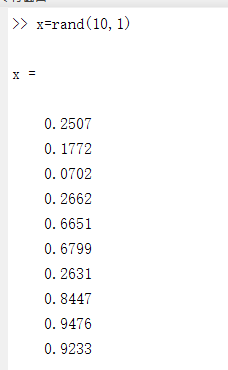## 2. 正态分布

### 概率密度曲线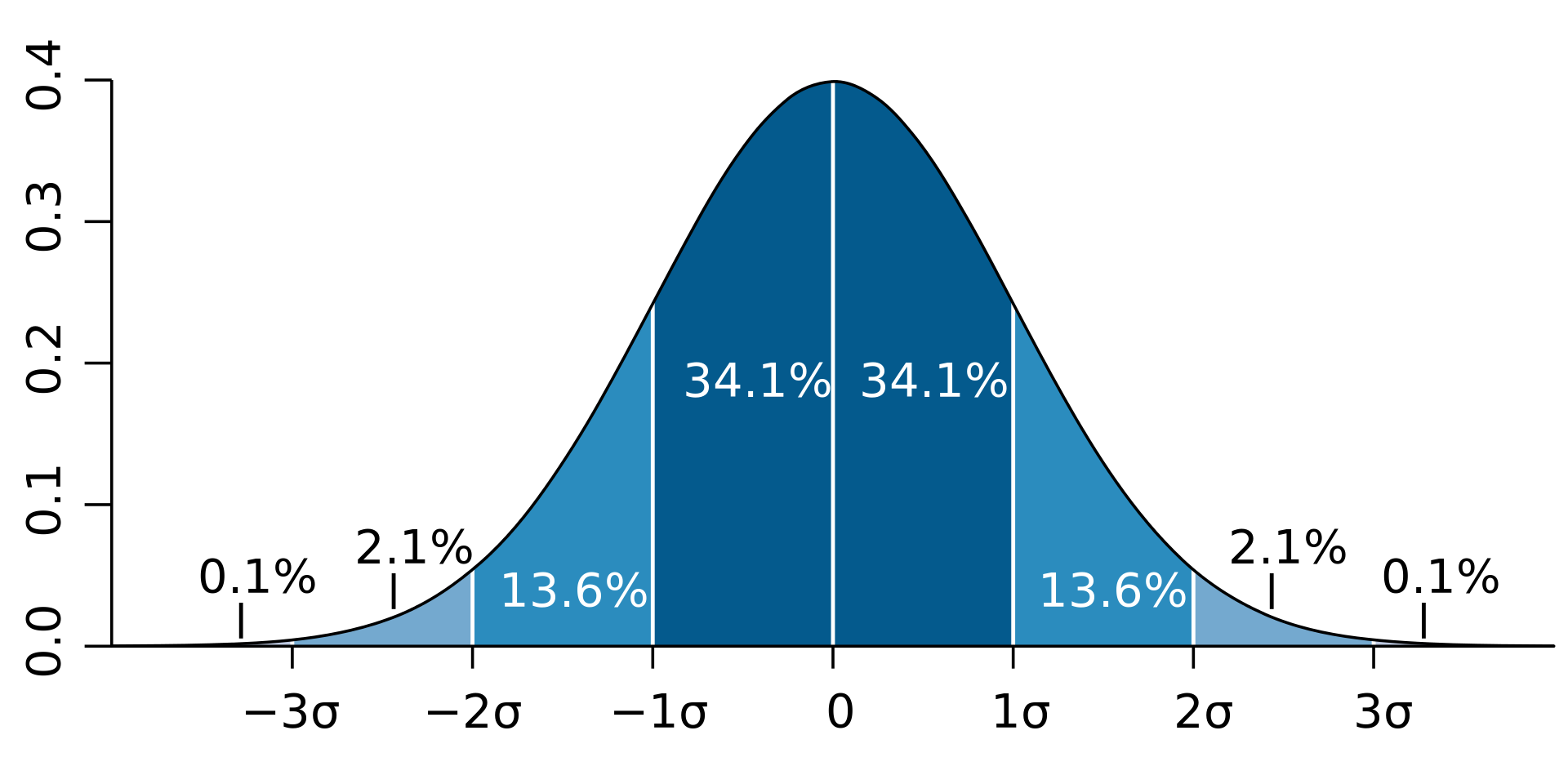### 2.1 标准正态分布

函数形式  x=randn(n,m)

• n - 行数
• m - 列数
• 生成均值为0，方差为1的标准正态分布

实例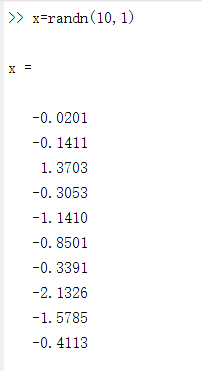### 2.2 设置均值，方差

函数形式：

•   - 均值
•  - 标准差
• a - 行数
• b - 列数
• 生成一个均值为，标准差为的正态分布随机数

实例：x=normrnd(3,10,[10,1])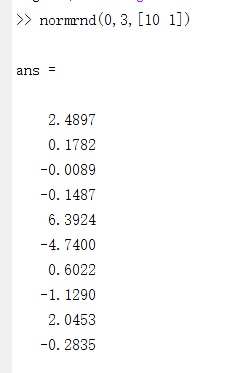## 3. 对数正态分布

注意：mu和sigma是对数正太分布的参数，不是数据本身的均值m和方差v。但两者存在如下关系：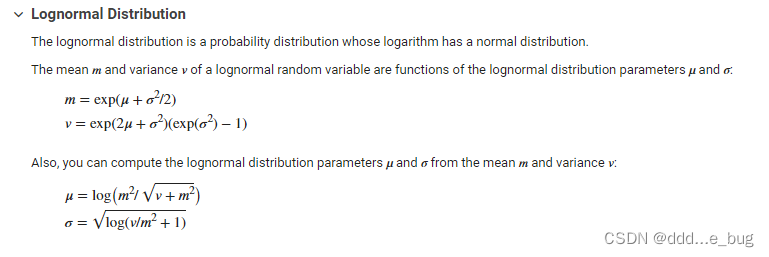• mu - 对数值的均值（mean of logarithmic values）；mu = log((m^2)/sqrt(v+m^2));  （m - 均值，v - 方差）
• sigma - 对数值的标准差（standard deviation of logarithmic values）；sigma = sqrt(log(v/(m^2)+1))
• a - 行数
• b - 列数

实例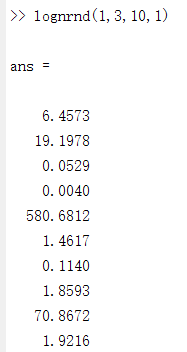展开全文开发语言
• 本代码主要利用MATLAB工具实现MATLAB——创建标准正态分布随机矩阵，简单明了，易于理解
• 本资源有两段代码，一段是用rand随机数循环算pi的值和矩阵算pi的值并进行对比和耗时...另一段是用matlab内置伪随机算法rand生成正态分布概率密度函数，并包含图像的生成和对比。有问题可以私信，有问必答，欢迎你的购买
• MATLAB如何使用normrnd函数生成正态分布随机数【语法说明】R=normrnd(mu,sigma)：生成服从参数为mu和sigma的正态分布的随机数。mu为均值，sigma为标准差。R是与mu、sigma同型的数组，如果mu和sigma之一为标量，则该...

MATLAB如何使用normrnd函数生成正态分布随机数

【语法说明】

R=normrnd(mu,sigma)：生成服从参数为mu和sigma的正态分布的随机数。mu为均值，sigma为标准差。R是与mu、sigma同型的数组，如果mu和sigma之一为标量，则该标量将被扩展为与另一个参数具有相同大小的数组。

R=normrnd(mu,sigma,m,n,…)：若N与P为标量，则函数按N和P的值生成m×n×……大小的随机数组R。如果N与P均不为标量，则必须满足[m, n, …]=size(N)，且N 与P 同型。

R=normrnd(mu,sigma,[m,n,…])：同R=normrnd(mu,sigma,m,n,…)。

【功能介绍】生成服从正态分布随机数。正态分布又称高斯分布，其特点是数据集中在平均值附近，离均值越远，出现的概率越小，曲线呈钟形。在正态分布中，mu为随机变量的期望，sigma为随机变量的标准差。正态分布的概率密度函数为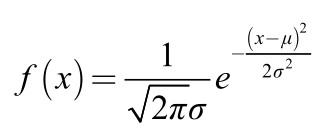【实例】生成10000个服从(3, 1)正态分布的随机数，并求随机数的均值与标准差。

>> a=normrnd(3,1,1,10000); % 生成服从(3, 1)正态分布的随机数

>> mean(a)　　　　　　% 求随机数均值

ans =

3.0050

>> std(a)　　　　　　　% 求随机数标准差

ans =

0.9897

【实例讲解】正态分布是一种连续型随机变量的概率分布，随机变量的取值可以为任意实数。正态分布是自然界中最常见的一种分布，在通信系统中常假设噪声服从正态分布，称高斯噪声。

展开全文• 文章目录代码mvnrnd输入参数mu——多元正态分布的均值sigma——多元正态分布的协方差n——多元随机数的个数mvnrnd输出参数R——多元正态随机数 代码 生成指定均值向量为(3, 2)，协方差矩阵为(11.51.54)\left(\begin...

# 代码

生成指定均值向量为(3, 2)，协方差矩阵为 ( 1 1.5 1.5 4 ) \left(\begin{aligned}&1&1.5\\&1.5&4\end{aligned}\right) 的二元正态分布的随机数：

mu = [3 2]; % 均指向量
nov = [1 1.5; 1.5 4]; % 协方差矩阵

% 生成100个二元正态分布随机数
R = mvnrnd(mu, nov, 100);

% 绘制二元正态分布散点图
scatter(R(:,1),R(:,2),'filled');
grid on;# mvnrnd输入参数

## mu——多元正态分布的均值

：数值向量 | 数值矩阵

含义：多元正态分布的平均值，指定为 1×d数值向量m×d数值矩阵。如果 mu 是一个向量，那么 mvnrd 复制该向量以匹配 sigma 的后续维度。如果 mu 是一个矩阵，那么 mu 的每一行就是单个多元正态分布的均值向量。

数据类型：整型 | 双精度浮点型

## sigma——多元正态分布的协方差

：对称半正定矩阵 | 数字数组

含义：多元正态分布的协方差，指定为 d×d对称半正定矩阵d×d×m 数值数组。如果 sigma 是一个矩阵，那么 mvnrnd 复制该矩阵以匹配 mu 中的行数。如果 sigma 是一个数组，那么 sigma 的每一个元素，sigma(: , :, i) 是单个多元正态分布的协方差矩阵，因此是一个对称的半正定矩阵。

如果协方差矩阵是对角矩阵，包含沿对角线的方差和零协方差，则还可以将 sigma 指定为 1×d向量 或 仅包含对角线项的1×d×m数组

数据类型：整型 | 双精度浮点型

## n——多元随机数的个数

：正标量整数

含义：多变量随机数的数目，指定为正标量整数。n 指定 R 中的行数。

数据类型：整型 | 双精度浮点型

# mvnrnd输出参数

## R——多元正态随机数

：数值矩阵

多元正态随机数，返回为以下值之一：

• mxd数值矩阵，其中 m 和 d 是由 μ 和 σ 指定的尺寸
• n×d数值矩阵，其中 n 是指定的输入参数，d 是由 mu 和 sigma 指定的维数

如果 mu 是矩阵，sigma 是数组，那么 mvnrnd 使用 mu(i, : ) 和 sigma(:, :, i) 计算 R(i, : )。

展开全文• 一种生成正态随机数的方法 生成步骤 第一步： 产生两个（0，1）上独立的均匀分布变量u1和u2 第二步：考虑如下两个变量 X1=(−2loge⁡(u1))1/2cos⁡(2πu2)" role="presentation" ...
• 即利用MATLAB软件的仿真功能来产生若干正态分布随机噪声,然后以数据文件的形式将其存储在EPROM中并在地址发生器作用下输出噪声,以定量检验直接序列扩频系统(DSSS)的抗干扰能力。最后给出了仿真信号波形和实验信号...
• 本博文源于matlab概率论内容。主要讲述正态分布的随机数绘制
• randMat：生成在指定区间 [a,b] 上具有均匀或正态分布的随机数矩阵，或者对于任意数量的 a,b 对具有特定的均值 (a) 和方差 (b)。 对于均匀分布，MATLAB 函数 rand() 返回的值乘以 (ba)，然后添加到 a。 对于正态分布...
• 调用normrnd函数生成1000行3列的随机数矩阵x，其元素服从均值为75，标准差为8的正态分布（1）代码（2）运行效果2. 调用normrnd函数生成1000行3列的随机数矩阵x，其各列元素分别服从不同的正态分布（1）代码（2）...
•c语言 开发语言 后端
• Date: 2018.8.5 转载自：... 功能：生成服从正态分布的随机数 语法： R＝normrnd(MU,SIGMA) R＝normrnd(MU,SIGMA,m) R＝normrnd(MU,SIGMA,m,n) 说 明： R＝normrnd(MU,SIG...
• 其中 为产生矩阵的行数和列， 为产生矩阵的个数，产生的矩阵中的每个元素均为服从 N(0,1)N(0, 1)N(0,1) 的标准正态分布的元素。使用如下命令产生一个 的服从的随机数矩阵： 绘制图像效果如下： 可以看到这里随机数...
• normrnd_normfit 帮助用户生成一个正态分布随机集数据，然后在数据的顶部和后面拟合高斯曲线计算其均值和标准差。 它可以帮助用户检查 NORMRND 函数完成了它的工作。它绘制了原始直方图和拟合的直方图。 normrnd_...
• 产生一组正态分布N（μ,σ^2）的高斯随机数，其样本个为N，估计该序列的均值、方差和相关函数。（1）互相关函数xcorr用法c=xcorr(x,y)；C=xcorr(x)a=normrnd(1,2,1,1000);c=xcorr(x)%计算自相关函数。title('自...算法 开发语言
• 此函数生成根据截断的正态分布（或通过转换为具有正支持的正态分布）分布的随机变量。 这种问题对于用 MCMC 方法生成变量特别有趣。 我们使用混合接受-拒绝算法，即经典的接受-拒绝算法使用多个提议分布，每个提议...
• 如何用均匀分布随机数生成正态分布随机数_doublehhcc的博客-CSDN博客_用均匀分布生成正态分布的随机数利用独立同分布的中心极限定理生成正态分布的随机数_m0_37772174的博客-CSDN博客_中心极限定理生成正态分布 ...
• 当x和y是两个独立且服从(0,1)均匀分布随机变量时，则 Z1=cos(2πx)⋅–2ln(1–y)−−−−−−−−−√Z1=cos⁡(2πx)⋅–2ln⁡(1–y)Z_1=\cos (2\pi x) \cdot \sqrt { – 2\ln (1 – y)}Z2=sin(2πx)⋅–2ln(1...随机数
• 使用 C 语言产生正态分布的随机数。给出了全部的源代码。产生的随机数保存在文本文件中，并给出了 MATLAB 直方图验证的源代码。
• 02我们打开matlab之后，点击菜单栏里的“importdata”,准备加载我们需要统计分析的数据。03打开加载界面之后，我们找到我们要加载的数据文件，点击打开。04在加载的界面，我们将类型选择“matrix”矩阵列表，选择...
• MATLAB产生正态分布随机噪声来测量直扩系统抗噪性能目前，扩频技术因其良好的抗干扰能力及低截获概率性，因而在通信领域得到了广泛的应用。直接序列扩频是扩频技术的一种主要方式，它是用PN码和二元信息数字序列模...
• 题目是 利用均匀分布 U（–1，1）,使用中心极限定理产生正态分布的随机数1000个,其中每次用于产生正态分布随机数的均匀分布样本容量 n >50
• R语言使用rnorm函数生成正态分布随机数、自定义指定生成随机数的个数、均值、方差r语言 数据挖掘 机器学习 人工智能
• 1.语法X = randnX = randn(n)X = randn(sz1,…,szN)X = ...说明X = randn 返回一个从标准正态分布中得到的随机标量。X = randn(n) 返回由正态分布的随机数组成的 n×n 矩阵。X = randn(sz1,…,szN) 返回由随机数...
• 正态分布是normpdf(x,mu,sigma)mu sigma 默认是 0 1例子ez由热心网友提供的答案1：%生成一组随机数(正态分布)data=normrnd(0,1,1,500);%绘制直方图hist(df=normrnd(0,1,1,100)hist(f)1、双击matlab软件图标，打开...
• randn 产生标准正态分布 N(0,1) 如果你想生成均值为a，方差为b的非标准正态分布N(a,b)，则为：a+b*randn(m,n)。其中：m为行数，n为列数。...产生对数正态分布随机数 mvnrnd 产生多元正态分布随机数 ...开发语言...

# matlab正态分布随机数matlab 订阅